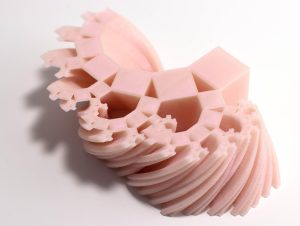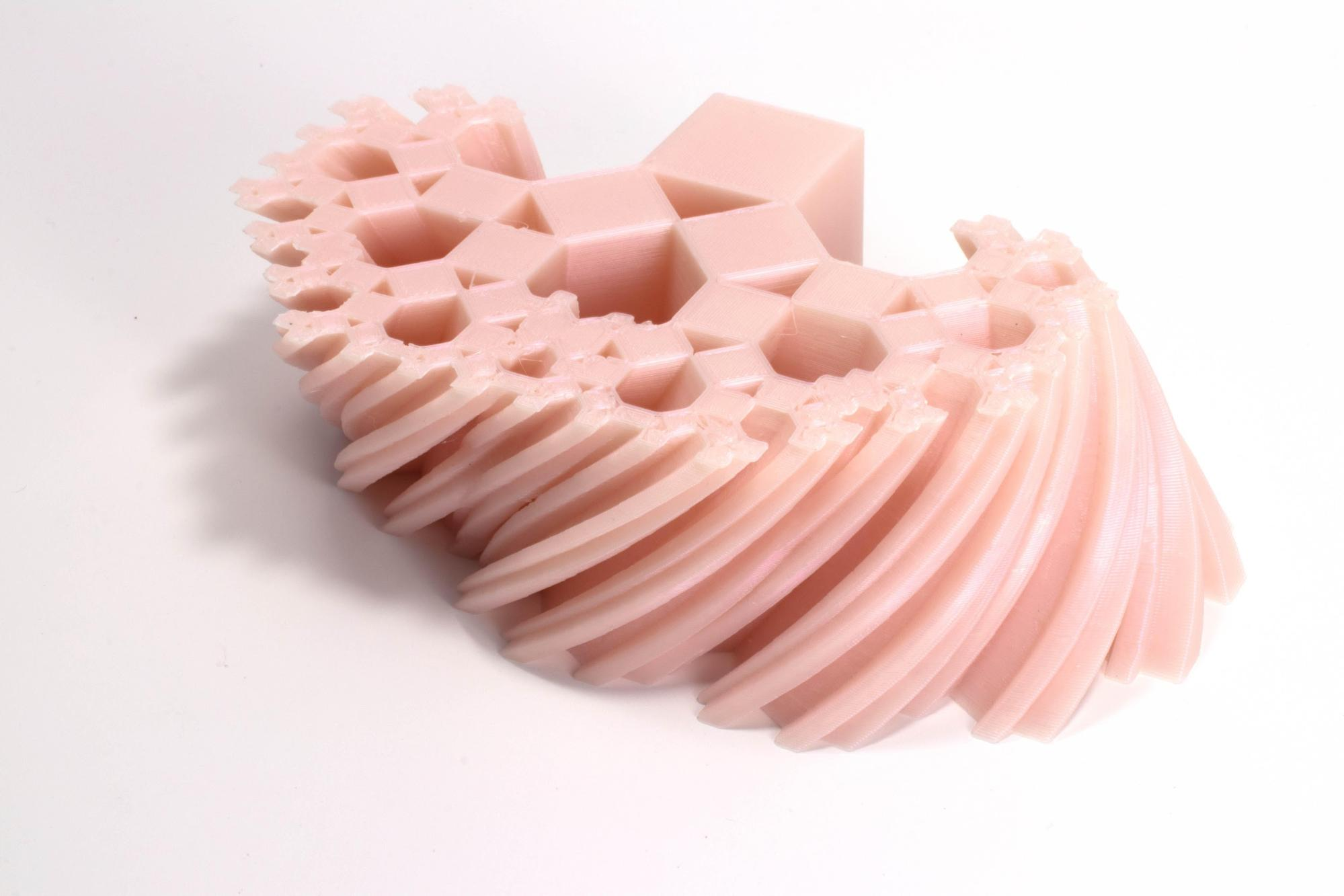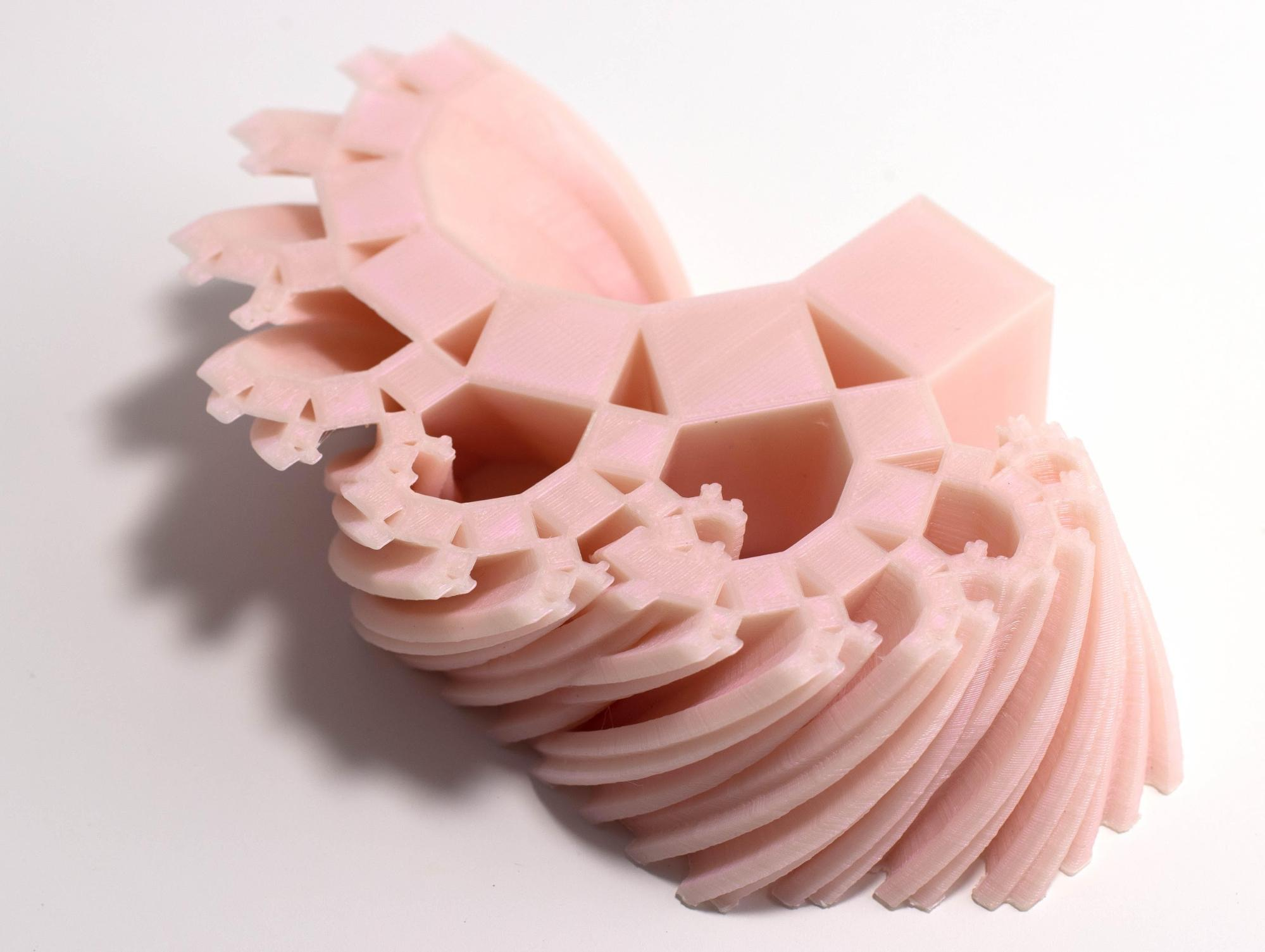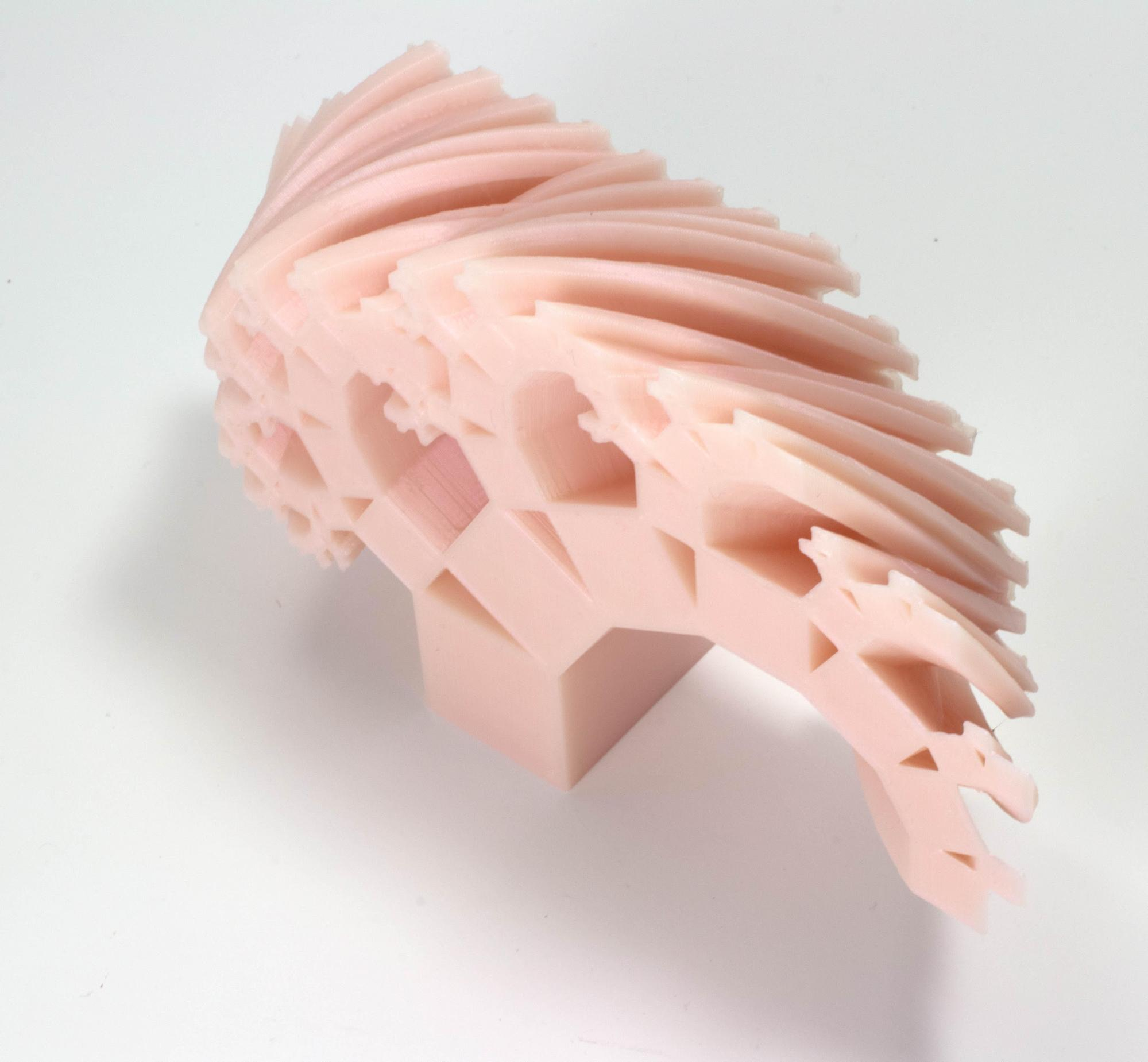# Deformation of the Pythagorean Tree Fractal

Date started: Fall 2017
Leads: Gabriel Dorfsman-Hopkins

###Abstract

The construction of the Pythagorean tree fractal is based on the choice of a right triangle (up to scaling). Therefore, really on the choice of a single acute angle. As you vary this angle, the associated fractal varies continuously (with a 45 degree angle giving the most familiar symmetric incarnation of the fractal). We vary the angle from 45 degrees to 60 degrees, 3d printing one layer for every tenth of a degree shift, capturing what is more commonly representing with an animation into a static 3 dimensional model. The model is built in OpenSCAD.

### Media